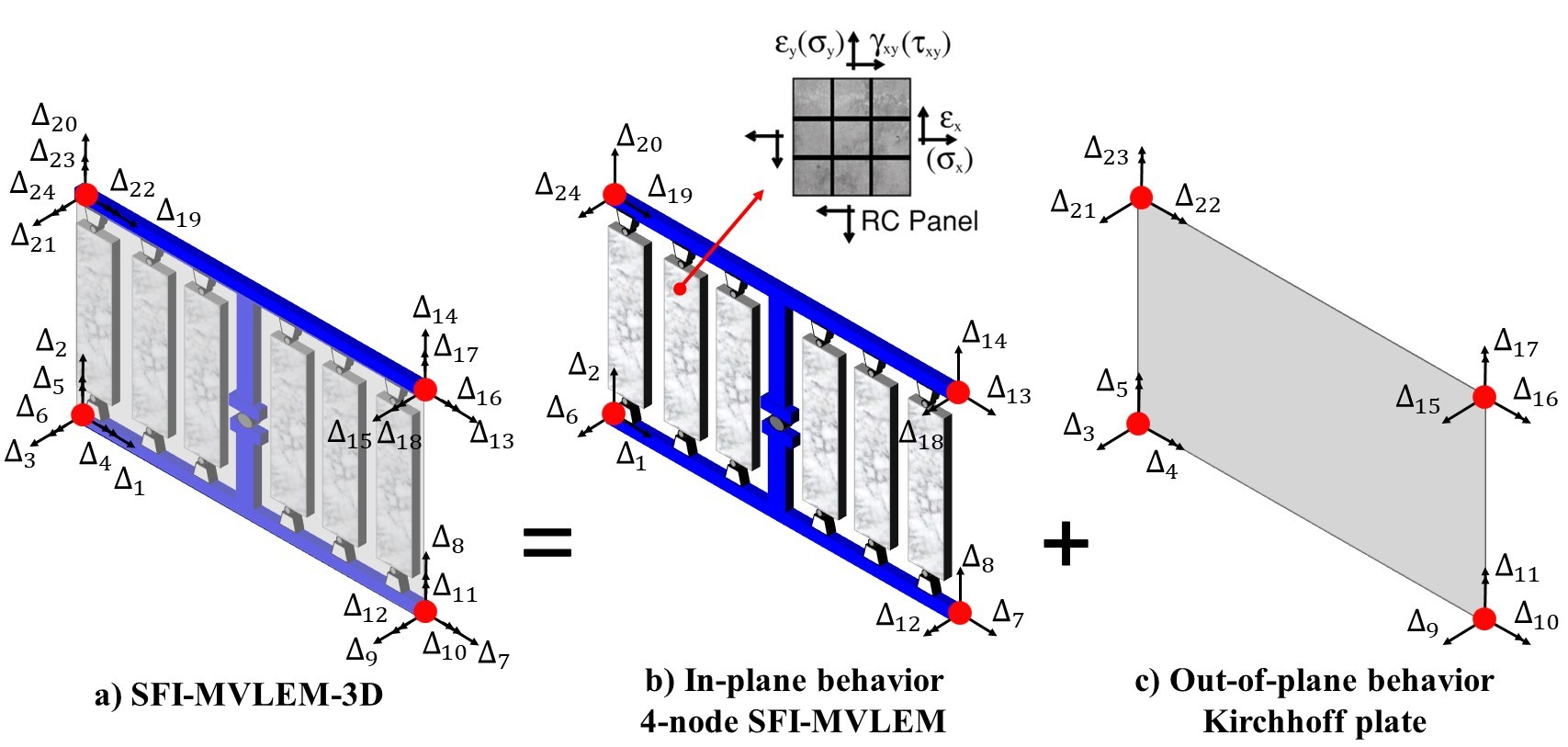# 4.2.7.12. SFI_MVLEM_3D - 3-D Shear-Flexure-Interaction Element for RC Walls¶

Developed and implemented by:
Kristijan Kolozvari (CSU Fullerton)
Kamiar Kalbasi (CSU Fullerton)
Kutay Orakcal (Bogazici University)
John Wallace (UCLA)

The SFI-MVLEM-3D model (Figure 1a) is a three-dimensional four-node element with 24 DOFs that incorporates axial-flexural-shear interaction and can be used for nonlinear analysis of non-rectangular reinforced concrete walls subjected to multidirectional loading. The SFI-MVLEM-3D model is an extension of the two-dimensional, two-node Shear-Flexure-Interaction Multiple-Vertical-Line-Element-Model (SFI-MVLEM). The baseline SFI-MVLEM, which is essentially a line element for rectangular walls subjected to in-plane loading, is extended in this study to a three-dimensional model formulation by applying geometric transformation of the element degrees of freedom that converted it into a four-node element formulation (Figure 1b), as well as by incorporating linear elastic out-of-plane behavior based on the Kirchhoff plate theory (Figure 1c). The in-plane and the out-of-plane element behaviors are uncoupled in the present model.

This element shall be used in Domain defined with -ndm 3 -ndf 6.Figure 1: SFI_MVLEM_3D Element Formulation

element('SFI_MVLEM_3D', eleTag, *eleNodes, m, '-thick', *thicks, '-width', *widths, '-mat', *matTags, <'-CoR', c>, <'-ThickMod', tMod>, <'-Poisson', Nu>, <'-Density', Dens>)
 eleTag (int) unique element object tag eleNodes (list (int)) a list of four element nodes defined in the counter-clockwise direction m (int) number of element uniaxial fibers thicks (list (float)) a list of m macro-fiber thicknesses widths (list (float)) a list of m macro-fiber widths matTags (list (int)) a list of m material tags corresponding to nDmaterial FSAM c (float) location of center of rotation from the base (optional; default = 0.4 (recommended)) tMod (float) thickness multiplier (optional; default = 0.63 equivalent to 0.25Ig for out-of-plane bending) Nu (float) Poisson ratio for out-of-plane bending (optional; default = 0.25) Dens (float) density (optional; default = 0.0)